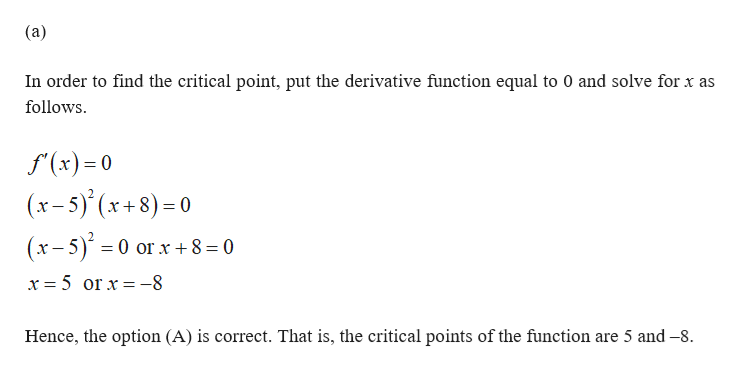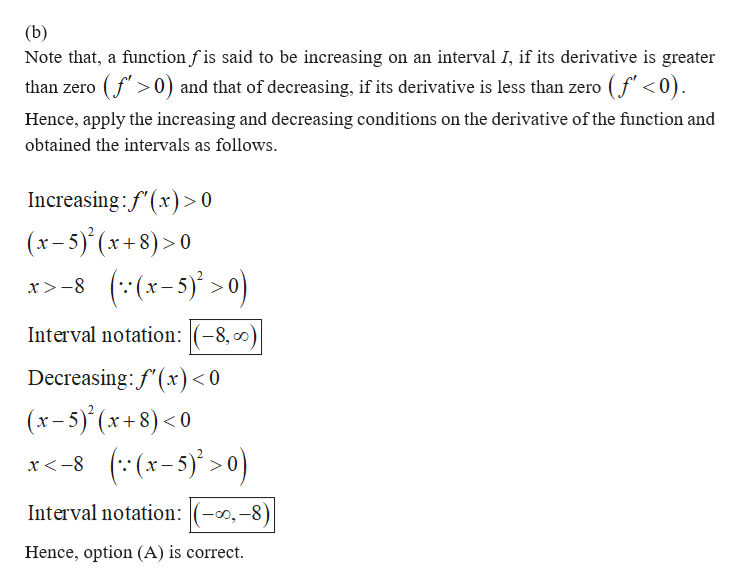Question
16 views

Can you help me with this problem step by step?

check_circle

Step 1help_outlineImage Transcriptionclose(a) In order to find the critical point, put the derivative function equal to 0 and solve for x as follows f(x)0 (x-5)(x+8)=0 (x-5)0 or x +8 = 0 x 5 or x-8 Hence, the option (A) is correct. That is, the critical points of the function are 5 and -8 fullscreen
Step 2help_outlineImage Transcriptionclose(b) Note that, a function f is said to be increasing on an interval I, if its derivative is greater than zero (f0) and that of decreasing, if its derivative is less than zero (f <0). Hence, apply the increasing and decreasing conditions on the derivative of the function and obtained the intervals as follows. Increasing:f'(x)> 0 (x-5)'(x+8)>0 x8(x-5)0 Interval notation:(-8, 0 Decreasing: f'(x < 0 (x-5)(x+8)<0 x-8 (x-5)>0) Interval notation:(-0,-8) Hence, option (A) is correct fullscreen

### Want to see the full answer?

See Solution

#### Want to see this answer and more?

Solutions are written by subject experts who are available 24/7. Questions are typically answered within 1 hour.*

See Solution
*Response times may vary by subject and question.
Tagged in

### Other